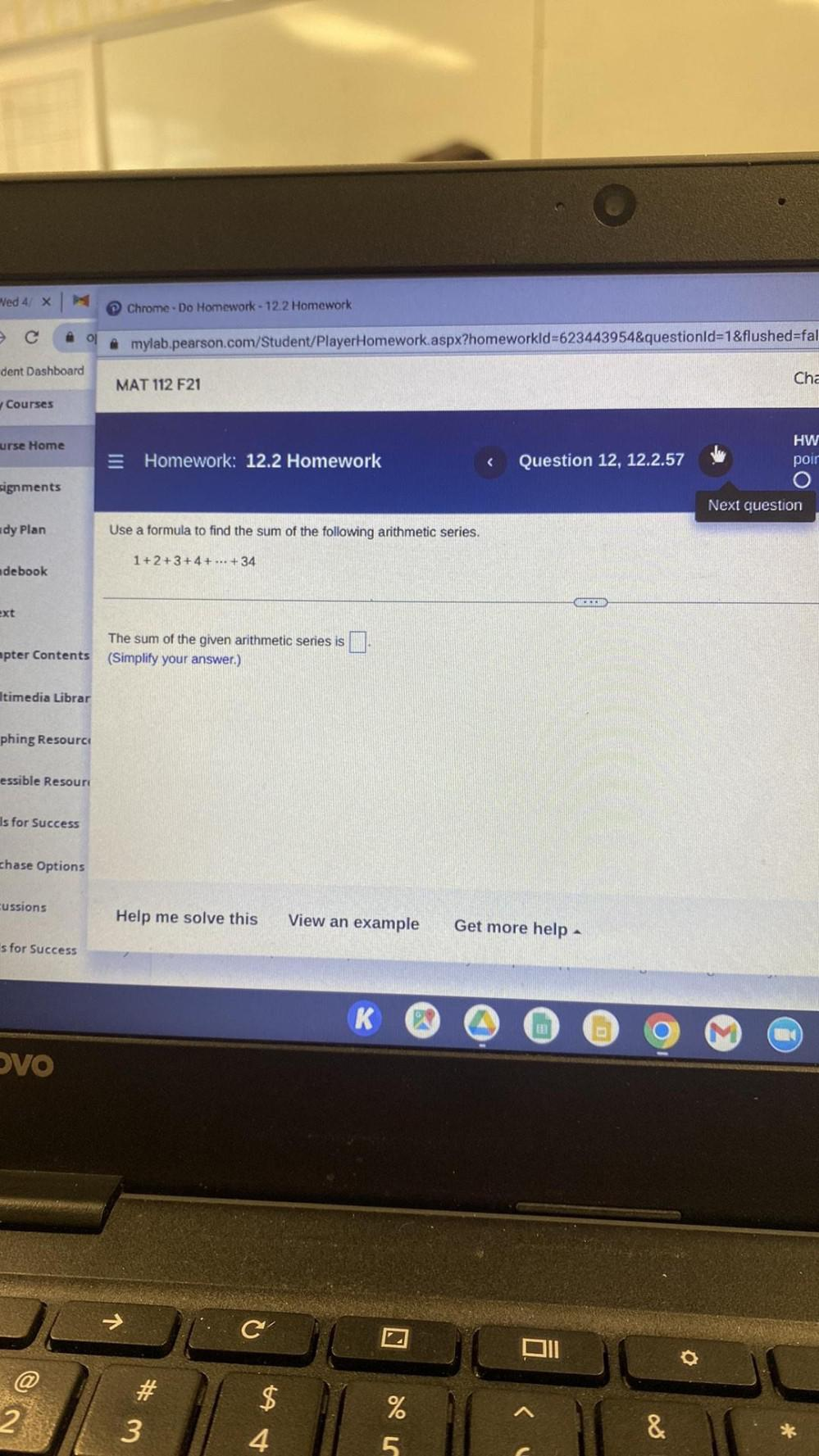Question:

# Use a formula to find the sum of the following arithmetic

Last updated: 7/26/2022Use a formula to find the sum of the following arithmetic series. 1+2+3+4+ ... +34 The sum of the given arithmetic series is (Simplify your answer)